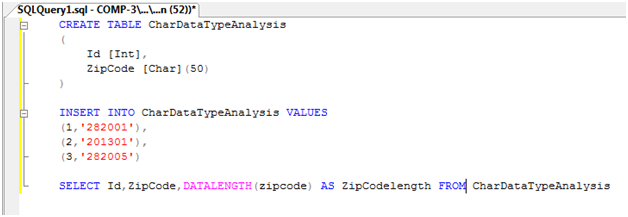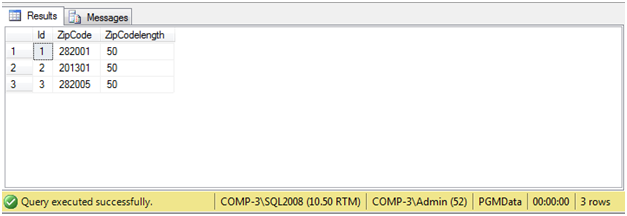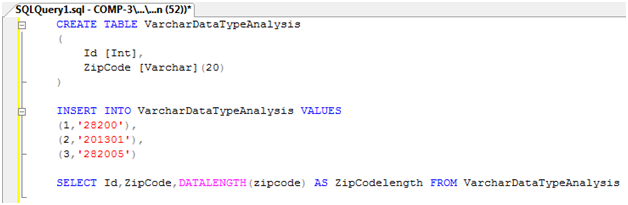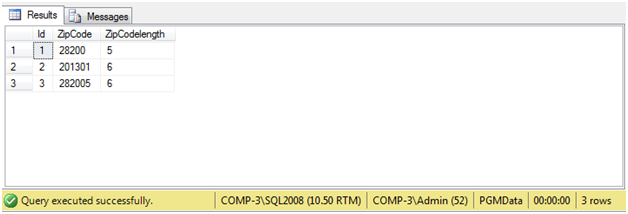## Thursday, May 22, 2014

### Difference between Char, Varchar, and nVarchar data type in SQL Server

In real world everyone has different need and requirement, why we use the same data type to store different types of value. What is the actual difference between Char, Varchar and nVarchar data type.

Char DataType
Char datatype which is used to store the fixed length, non-unicode characters data. Suppose if we declared char (50) it will allocates memory for 50 characters. Once we declare char (50) and if we enter fewer than the number of characters defined (i.e. 6), the remaining length will be space filled to the right. it means other 44 characters of memory will be wasted.

Now we look in the query, How will it effect.Output of this Select Statement:Use this data type when the column data is to be of fixed length, which tends to be the case
for customer IDs and bank account IDs.

Nchar DataType
Nchar type is exactly similar to char. But hold character in Unicode format rather than ANSI. The Unicode format has a larger character set range than ANSI. Unicode datatype take exact double memory space in sql server. So use the nchar when you want to store the Unicode value.

varchar DataType

Varchar datatype, which is used to store the alphanumeric values, just like char datatype. If both are similar than what is the difference between char and Varchar? What is the need to create the new datatype? Later in this article we will discuss this. The maximum size of a Varchar column is 8,000 characters. However, if you define the column with no size—that is, Varchar () — then the length will default to 1.

Difference between the char and Varchar datatype

Char belongs to fixed length characters datatype, Varchar belongs to variable length characters datatype. If you define a column to be 20 characters long, but If you enter fewer than the number of characters defined like 10 characters then it will consume only 10 characters memory. It will not consume the defined memory.

Now we look in the query:Output of the select statement:We can see in above image the output of the select statement, the ZipCodelength value are varying according to data in zipCode field.

So we should use the Char for the fixed length data field and Varchar for the variable length data field.

Nvarchar DataType

Nvarchar datatype same as varchar datatype but only difference nvarchar is used to store Unicode characters and it allows you to store multiple languages in database. nvarchar datatype will take twice as much space to store extended set of characters as required by other languages.

Difference between Varchar and Nvarchar DataType
 Varchar(n) Nvarchar(n) 1.    Non-Unicode Variable Length character data type. Example: Declare @FirstName As Varchar(20) = ‘Developer’ Select @FirstName 2.    It takes 1 byte per character. Example: Declare @FirstName As Varchar(20) = ‘Developer’ Select @FirstName AS FirstName, DATALENGTH(@FirstName) As Length Result: FirstName Length Developer 9 3.    Can store maximum 8000 Non-Unicode characters. 4.    If the value of n is not specified at the time of variable declaration or column definition then it is considered as 1. Example: Declare @FirstName As Varchar =’Developer’ SELECT @firstName FirstName, DATALENGTH(@firstName) Length Result: FirstName Length D                1 5.     If n is not specified in while using Cast/Convert   functions, then it is considered as 30.      Example:      Declare @firstName Varchar(50)=‘Unicode character sets hold up to 65,536’    Select CAST(@firstName As Varchar) FirstName,DATALENGTH(CAST(@firstName As varchar)) Length        Result:FirstName                                LengthUnicode character sets hold up  30 1.    Unicode Variable Length character data type. Nvarchar  can store both the Unicode and non-Unicode (i.e) Chinese, Japanese, Korean etc) character. Example: Declare @FirstName As Nvarchar(20) = ‘Developer’ Select @FirstName 2.    It takes exactly double bytes per character as compare to Varchar. It takes 2 bytes per Unicode/Non-Unicode character. Example: Declare @FirstName As Nvarchar(20)= ‘Developer Select @FirstName AS FirstName, DATALENGTH(@FirstName) AS Length Result: FirstName Length Developer 18 3.    Can store maximum 4000 Unicode/Non-Unicode characters. 4.    If the value of n is not specified at the time of variable declaration or column definition then it is considered as 1. Example: Declare @FirstName As Nvarchar =’Developer’ SELECT @firstName FirstName, DATALENGTH(@firstName) Length Result: FirstName Length D                2 5.    If n is not specified in while using Cast/Convert     functions, then it is considered as 30.       Example:        Declare @firstName Nvarchar(50)= ‘Unicode character sets hold up to 65,536’     Select CAST(@firstName As Nvarchar) FirstName, DATALENGTH(CAST(@firstName As Nvarchar)) Length    Result: FirstName                                 Length Unicode character sets hold up   60

So if you are not using other languages then it’s better to use varchar datatype instead of nvarchar.